# Test: Previous Year Questions: Solutions

## 26 Questions MCQ Test Topic-wise MCQ Tests for NEET | Test: Previous Year Questions: Solutions

Description
Attempt Test: Previous Year Questions: Solutions | 26 questions in 35 minutes | Mock test for NEET preparation | Free important questions MCQ to study Topic-wise MCQ Tests for NEET for NEET Exam | Download free PDF with solutions
QUESTION: 1

### Which of the following concentration factor is affected by change in temperature ?  [AIEEE-2002]

Solution:

Molarity is temperature dependent. In the formula of molarity, you have L of solution. Volume is temperature-dependent. It varies with changing temperature. Because of this, molarity changes if the temp changes.

QUESTION: 2

### For an aqueous solution, freezing point is _0.186ºC . Boiling point of the same solution is (Kƒ = 1.86º K mol-1 kg) and Kb = 0.512º K mol-1 kg) [AIEEE-2002]

Solution:

ΔTb/Kb = ΔTf/Kf
0.186/18.6 = ΔTb/0.512
ΔTb = 0.1x0.512
= 0.0512.
Boiling point = boiling point (of water) + ΔTb
=> 100 + 0.0512
= 100.0512

QUESTION: 3

### In a mixture of A and B, components show negative deviation when [AIEEE-2002]

Solution:

The correct answer is option A.
Negative deviation means lower vapour pressure, it suggests high boiling point, thus resultant intermolecular force should be stronger than individual one.

QUESTION: 4

A pressure cooker reduces cooking time for food because _

[AIEEE-2003]

Solution:
Option D is correct To explain this, first of all I would like to define boiling point- the temp. at which vapour pressure of liquid becomes equal to atmospheric pressure, called B. P.
when we close the cooker then pressure inside the coocker increases as compared to normal atmosphereic pressure, then if we start heating then it will not boils at 100 degree centigrade( as we see in normal cases under atmosphereic pressure) becz the pressure inside the cooker is higher. So it need more temp. to boil that's why at higher temp cooking time reduces.
QUESTION: 5

If liquids A and B form an ideal solution _

[AIEEE-2003]

Solution:

The correct answer is option C
If liquids A and B form an ideal solution, the enthalpy of mixing is zero. For an ideal solution ΔVmixing​=0, ΔHmixing​=0. The Gibbs free energy is always negative and becomes more negative as the temperature is increased.

QUESTION: 6

In a 0.2 molal aqueous solution of a weak acid HX the degree of ionization is 0.3 . Taking kƒ for water as 1.85, the freezing point of the solution will be nearest to _

[AIEEE-2003]

Solution:

The correct answer is option C
ΔT = Kf​ × molality × (1+α)(HX ⇌ H+   + X−)
Given molality = 0.2;  degree of ionization is 0.3
=1.85×0.2×(1+0.3)
=0.481K
The freezing point of the solution will be 0.81 K.

QUESTION: 7

If liquid A and B form ideal solution, than

[AIEEE-2003]

Solution:

The correct answer is Option B.
If liquids A and B form an ideal solution, the enthalpy of mixing is zero.
For ideal solution ΔVmixing = 0, ΔHmixing = 0. The Gibbs free energy is always negative and becomes more negative as the temperature is increased.

QUESTION: 8

Which one of the following aqueous solutions will exhibit highest boiling point ?

[AIEEE-2004]

Solution:

The correct answer is Option A.
Greater the number of solute particles in the solution, higher the elevation in boiling point. Among the given alternatives, Na2SO4 will exhibit the highest boiling point since it will dissociate into 3 ions when dissolved in the solution.

QUESTION: 9

Osmotic pressure of 40% (wt./vol.) urea solution is 1.64 atm and that of 3.42% (wt./vol.) cane sugar is 2.46 atm. When equal volumes of the above two solutions are mixed, the osmotic pressure of the resulting solution is -

Solution: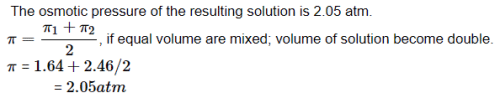QUESTION: 10

Which of the following liquid pairs shows a positive deviation from Raoult's law ?

[AIEEE-2004]

Solution:

The correct answer is option B
Benzene - methanol shows a positive deviation from Raoult's law.
Benzene reduces H -bonding between methanol molecules and thus, evaporation increases or boiling point decreases, i.e., positive deviation from Raoult's law.Thus  in benzene - methanol mixture the intermolecular forces of attractions are weaker than those in pure liquids.

QUESTION: 11

Which one of the following statement is false?

[AIEEE-2004]

Solution:

The correct answer is option D
The statement D is false.
Two sucrose solutions of the same molality prepared in different solvents will have different freezing point depression. This is because the molal depression in freezing point constant Kb​ is different for different solvents.
The freezing point depression = ΔTf​=Kb​×m
Here, m is the molality of sucrose solution.

QUESTION: 12

If a is the degree of dissociation of Na2SO4, the vant Hoff's factor (i) used for calculating the molecular mass is–

[AIEEE-2005]

Solution:

The correct answer is option D
For Na2​SO4 ​: −i = n
Na2​SO4 ​⟶ 2Na+ + SO42−​​
n=2
i=1−α+nα
i=1−α+2α
i=1+2α

QUESTION: 13

Benzene and toluene form nearly ideal solutions. At 20ºC, the vapour pressure of benzene is 75 torr and that of toluene is 22 torr. The partial pressure of benzene at 20ºC for a solution containing 78 g of benzene and 46 g of toluene in torr is

[AIEEE-2005]

Solution:

The correct answer is Option B.

The molar mass of benzene is 78 g

The molar mass of toluene is 92 g

nBen = 78/78 =1

nTol = 92/46 = 0⋅5

XBen = 1 / 1+0⋅5

= 1⋅5

PBen = XBenP0Ben
= (1/1⋅5) × 75
=​  50

QUESTION: 14

Two solutions of a substance (non electrolyte) are mixed in the following manner. 480 ml of 1.5 M first solution + 520 mL of 1.2 M second solution. What is the molarity of the final mixture ?

[AIEEE-2005]

Solution:

The correct answer is option D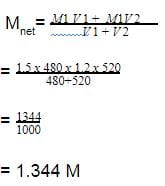QUESTION: 15

Which one of the following aqueous solutions will exhibit highest boiling point?

Solution:

The correct answer is option C
Greater the number of solute particles in the solution, higher the elevation in boiling point. Among the given alternatives, Na2​SO4​ will exhibit the highest boiling point since it will dissociate into 3 ions when dissolved in the solution.
△ = i × kb​×m
i × m of Na2​SO4​ is highest, hence its boiling point will also be highest.
Na2​SO4​         i × m=3×0.01=0.03
KNO3​            i × m=2×0.01=0.02
Urea             i×m=1×0.015=0.015
Glucose       i×m=1×0.015=0.015

QUESTION: 16

18 g of glucose (C6H12O6) is added to 178.2 g of water. The vapour pressure of water for this aqueous solution at 100º C is -

[AIEEE 2006]

Solution:

The correct answer is Option C.

The molar masses of glucose and water are 180 g/mol and 18 g/mol respectively.

Number of moles of glucose = 18/80 = 0.1
Number of moles of water = 178.2/18
=  9.9
Mole fraction of glucose = 0.1/(0.1+9.9)
= 0.01
Relative lowering in vapour pressure is equal to the mole fraction of glucose.
(760−P)/760 = 0.01
760 - P = 7.6
P = 760 −7.6 = 752.4 torr

QUESTION: 17

Density of a 2.05 M solution of acetic acid in water is 1.02 g/mL. The molality of the solution is -

[AIEEE 2006]

Solution:

The correct answer is option B
weight of acetic acid =2.05×60=123g2.05×60=123g
Weight of  solution =1000×1.02=1020g=1000×1.02=1020g
∴ Weight of water=(1020−123)=897g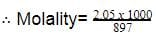=2.285 mol kg-1

QUESTION: 18

A mixture of ethyl alcohol and propyl alcohol has a vapour pressure of 290 mm at 300 K. The vapour pressure of propyl alcohol is 200 mm. If the mole fraction of ethyl alcohol is 0.6, its vapour pressure (in mm) at the same temperature will be -

[AIEEE 2007]

Solution:

The correct answer is Option A.

Total pressure =290 mm
Vapour pressure of propyl alcohol =200 mm
Mole fraction of ethyl alcohol = 0.6
Mol fraction of propyl alcohol =1− 0.6 = 0.4

PT = P0A × χA + P0B × (1−χA)
290 = 200 × 0.4 + PoB × 0.6
0.6 × P0B = 290 − 80
P0B = 350 mm

QUESTION: 19

A 5.25% solution of a substance is isotonic with a 1.5% solution of urea (molar mass = 60 g mol-1) in the same solvent. If the densities of both the solutions are assumed to be equal to 1.0 gcm-3, molar mass of the substance will be-

[AIEEE 2007]

Solution:

The correct answer is option D
For isotonic solutions, the osmotic pressure is the same. When solutions do not associate, Ⅱ = CRT and isotonic solutions will have the same concentration.
Let the molar mass of substance be M. It’s molar concentration will be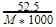The molar concentration of urea solution is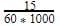According to question,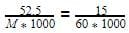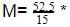60 = 210.0 g/mol.
Hence, the molar mass of the substance is 210.0 g/mol.

QUESTION: 20

The density (in g mL-1) of a 3.60 M sulphuric acid solution that is 29% H2SO4(Molar mass = 98 g mol-1) by mass will be -

[AIEEE 2007]

Solution:

The correct answer is Option C.

Mass % of H2SO4=29%
Therefore 100g solution contains 29g H2SO4

Let the density of solution (in g/ml) is d
Molarity of solution :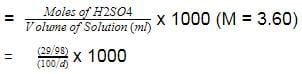QUESTION: 21

The vapour pressure of water at 20º C is 17.5 mm Hg. If 18g of glucose (C6H12O6) is added to 178.2 g of water at 20° C, the vapour pressure of the resulting solution will be –

[AIEEE 2008]

Solution:

The correct answer is option C
No. of moles of glucose=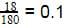No. of moles of water=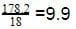Mole fraction of glucose=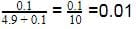Using Raoult's law, as solute is non-volatile,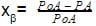PoA ​=vapour pressure of pure water
PA ​=vapour pressure of the solution
xβ​=mole fraction of glucose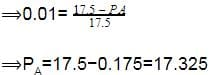The vapor pressure of the mixture=17.325 mm Hg.

QUESTION: 22

At 80º C , the vapour pressure of pure liquid `A' is 520 mm Hg and that of pure liquid `B' is 1000 mm Hg. If a mixture solution of `A' and `B' boils at 80º C and 1 atm pressure, the amount of `A' in the mixture is (1 atm = 760 mm Hg)

[AIEEE 2008]

Solution:

Let the amount of A in the mixture be χA​ and B be the χB​.
PT​= 760 =PAo​χA​ + PBo​χB
760=520χA​ + 1000(1−χA​)
480χA​=240
χA​= 1/2​ or 50%.

QUESTION: 23

A binary liquid solution is prepared by mixing n-heptane and ethanol. Which one of the following statements is correct regarding the behaviour of the solution ?

[AIEEE 2009]

Solution:

The correct answer is Option A.
n-Heptane is non-polar and ethanol is polar. Hence the mixture formed by these two liquids is non-ideal. The stronger forces of attraction between n-heptane-n-heptane and ethanol-ethanol are replaced by weaker forces of attraction between n-heptane-ethanol in the solution. Therefore the escaping tendency of the liquid molecules into the vapour phase increases. This will increase the vapour pressure more than expected from Raoult's law. This is a positive deviation.
Hence, the correct option is B

QUESTION: 24

Two liquids X and Y form an ideal solution At 300 K, vapour pressure of the solution containing 1 mol of X and 3 mol of Y is 550 mmHg. At the same temperature, if 1 mol of Y is further added to this solution, vapour pressure of the solution increases by 10 mmHg. Vapour pressure (in mmHg) of X and Y in their pure states will be, respectively -

[AIEEE 2009]

Solution:

The correct answer is option B
Let
P = xx​Px0 ​+ xy​Py0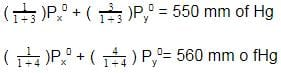on solving for Py0​ and Px0
We get,
Px0​ = 400 mm of Hg
Py0 ​= 600 mm of Hg

QUESTION: 25

Kf for water is 1.86 K kg mol-1. If your automobile radiator holds 1.0 kg of water, how many grams of ethylene glycol (C2H6O2) must you add to get the freezing point of the solution lowered to – 2.8°C ?

[AIEEE-2012]

Solution:

The correct answer is Option A.

Here ΔTf = 0−(−2.8oC)=2.8
∵ΔTf = Kf [W / (M1×W2)]
∵ 2.8 = 1.86 × W / (62×1)
⇒W = 93.33 ≈ 93 g

QUESTION: 26

The density of a solution prepared by dissolving 120 g of urea (mol. mass = 60 u) in 1000g of water is 1.15 g/mL. The molarity of this solution is :

[AIEEE-2012]

Solution:

The correct answer is option C
Molarity =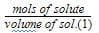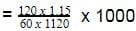=2.05 MUse Code STAYHOME200 and get INR 200 additional OFF Use Coupon Code